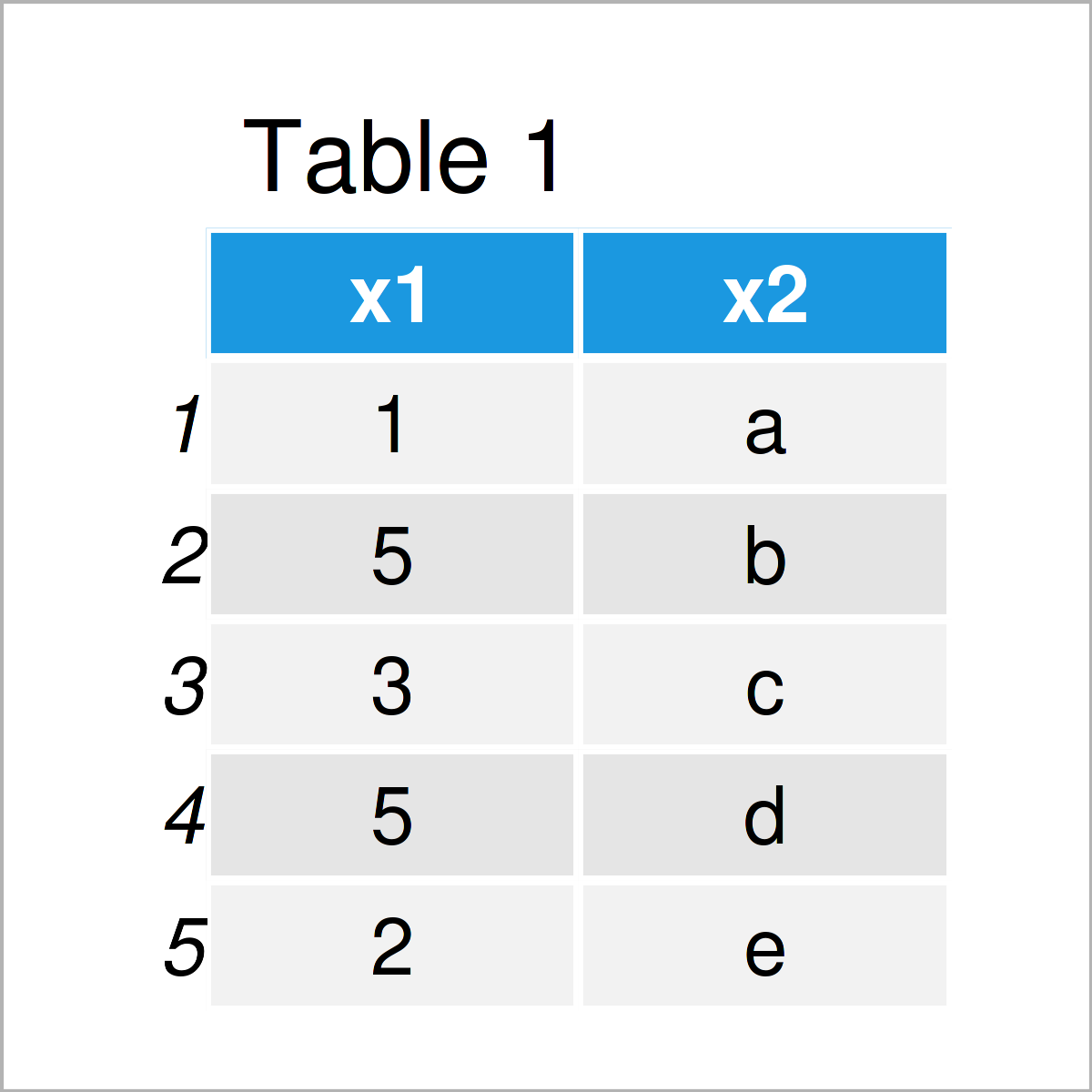# Calculate Difference Between Consecutive Rows in R (Example)

In this R programming tutorial you’ll learn how to find the difference of consecutive row values.

The article will consist of the following contents:

Let’s dig in…

## Creation of Example Data

The first step is to create some example data:

```data <- data.frame(x1 = c(1, 5, 3, 5, 2),    # Create example data frame
x2 = letters[1:5])
data                                         # Print example data frame```Have a look at the table that got returned after running the previous R programming syntax. It shows that the example data consists of five rows and two columns. The variable x1 has the numeric class and the variable x2 has the character class.

## Example: Get Difference Between Row Values Using diff() Function

In this example, I’ll explain how to compute the difference between subsequent rows in a data frame column.

For this task, we can apply the diff function to a particular data frame column (i.e. x1):

```diff_x1 <- diff(data\$x1)                     # Apply diff function
diff_x1                                      # Return row differences
#   4 -2  2 -3```

The previous output shows the result after subtracting subsequent row values.

## Video, Further Resources & Summary

Do you need further explanations on the R codes of this page? Then I recommend having a look at the following video on my YouTube channel. In the video, I show the R syntax of this article.

In addition, you might want to have a look at some of the other posts on my website:

In this R tutorial you have learned how to calculate the difference of consecutive rows. In case you have additional questions and/or comments, kindly let me know in the comments section below.

Subscribe to the Statistics Globe Newsletter